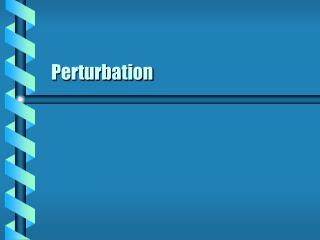DownloadDownload PresentationPerturbation

# Perturbation

Download Presentation## Perturbation

- - - - - - - - - - - - - - - - - - - - - - - - - - - E N D - - - - - - - - - - - - - - - - - - - - - - - - - - -
##### Presentation Transcript

1. Perturbation

2. Simple system with added effect. Basic Lagrangian L0 Perturbing term U Express as a perturbed Hamiltonian. Formed in the usual way Write as a first-order power series. l = 1 for perturbed motion Perturbed System

3. Time-independent systems can use J, w. Action-angle variables Unperturbed H0(J0) only Require a contact transformation for H(J) . Identity for l = 1 Find the action Stationary State

4. Power Series • The Hamiltonian can be expressed in l.

5. All dynamic variables are expressed as periodic functions of both old and new angle variables. Differ by a periodic function Unit period Terms are also periodic in old angles. Choose to have mean = 0 Periodic Variables

6. The mean value can be found for each term in the Hamiltonian Split V into average and oscillating term Can solve for S1, S2 Equating Terms

7. Perturbed Charge • Charge under two forces • Attractive Coulomb force • Uniform magnetic field • Let the magnetic field be a perturbation. Z Y X

8. The perturbing potential can be extracted from the Hamiltonian. Approximate A as small Find the average value of V. Use angular momentum l Or use action variable J Perturbing Potential

9. New Frequency • The perturbation is first order only. • Alter the frequency accordingly. next